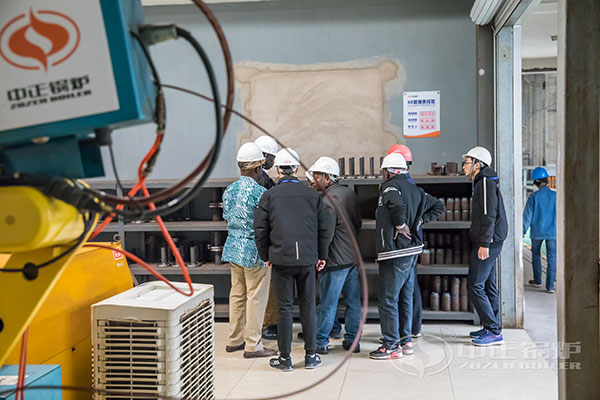# Boiler Steam Production Conversion Ton Hr to Kw

July 14, 2020

When discussing and purchasing boilers, people often use a few tons to indicate the size of the boiler. The first thing to explain is that the so-called few tons does not mean the weight of the boiler. It actually means “steamed tons”, which means the power of the boiler, the unit symbol is “t/h (steam ton per hour)”, which is the amount of steam that the boiler can produce per hour.Regarding the power unit, the well-known one usually uses more kw, so how many kw is 1 ton of boiler in the boiler unit?
According to accurate conversion, 1t/Hr=751.88kw, that is, the so-called 1 ton boiler in the boiler is equal to 751.88kw, which is approximately equal to 750kw. In addition, 1t/Hr=646500Kcal/Hr, that is to say, 1 ton boiler does not count the loss The heat output per hour is 646,500 kcal, which is approximately equal to the heat of 600,000 kcal.

European and American steam boilers are often marked with the word “at 212”, which means that the amount of evaporation refers to the evaporation of 212 degrees Fahrenheit water to 212 degrees Fahrenheit? That is, the evaporation of 100 ℃ water to 100 ℃ steam . In this way, 1kg evaporation is equivalent to 540kcal heat, we call it “equivalent evaporation”, that is: 1T/h = 540,000kcal/h. It can also be deduced from this that the relationship between boiler horsepower and “equivalent evaporation” is: 1BHp = 15.62kg/h.

1. Boiler evaporation and boiler thermal efficiency 1 ton/hour (t/h) ≈60×104 kilocalories (calories)/hour (kcal/h) ≈0.7 megawatts (MW) ≈720K kilowatts (KW)
2. Boiler evaporation and boiler horsepower 1 ton/hour (t/h) ≈ 71.1 boiler horsepower (BHP)
3. Boiler pressure engineering unit and international measurement unit 1 megapascal (Mpa) ≈10 kgf/cm2 (kgf/cm2)
4. Megapascals and Pascals 1 Megapascal (Mpa) = 106 Pascals (pa) 1 Pascal (pa) = 0.01mbar (mbar) ≈10-5 kgf/cm2 (engineering atmospheric pressure) (kgf/cm2) 1 Pa (Pa) ≈0.1 mm water column (mmH2O)
5. Force and gravity 1 kilogram force (kgf) = 9.81 Newton (N)
6. Heat 1 kilocalorie (kcal) = 4.187 kilojoules (KJ) 7. Volume (volume) volume 1 cubic meter (m3) = 1000 liters (L) 1 liter (L) = 1000 milliliters (ML)